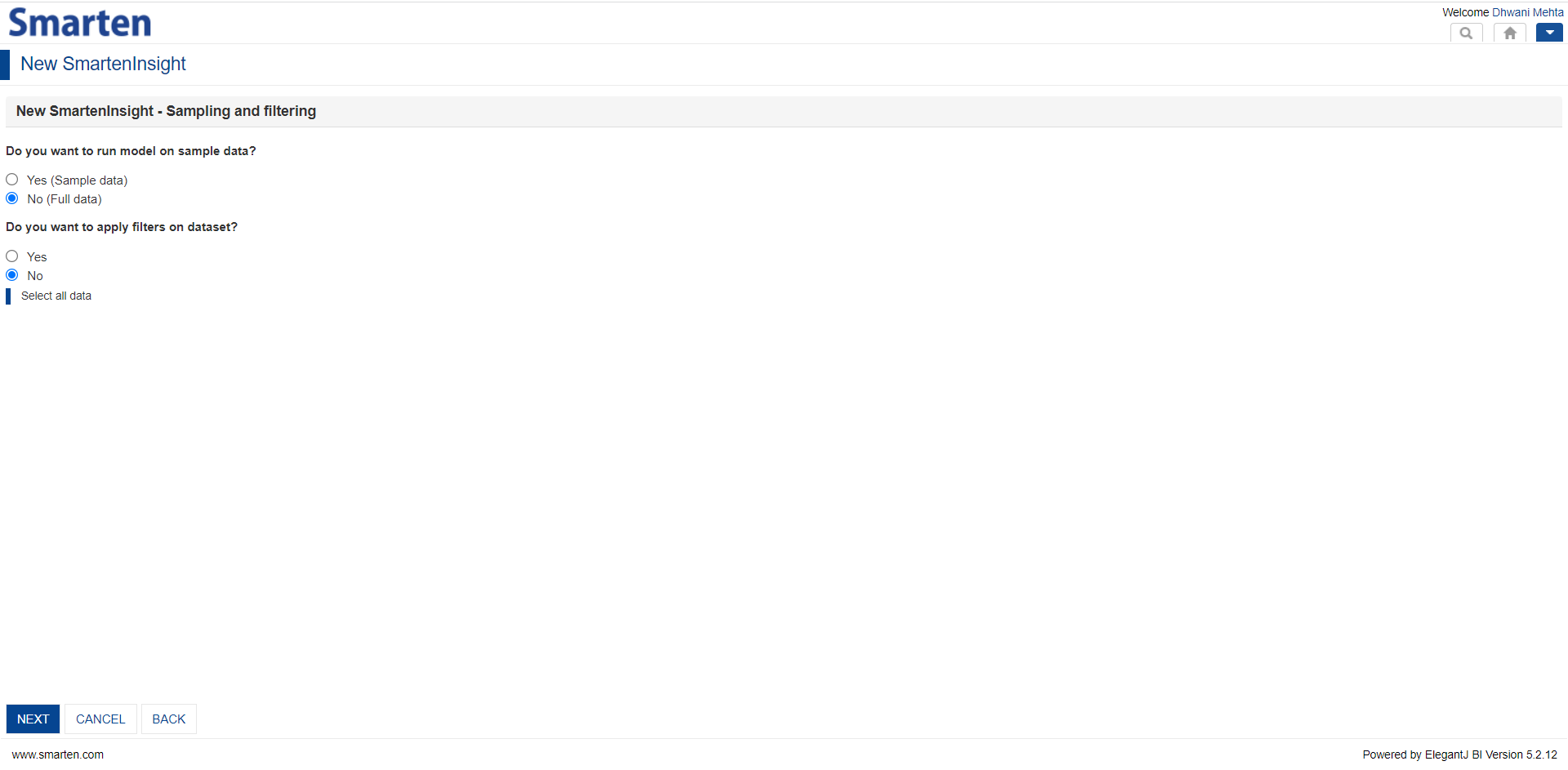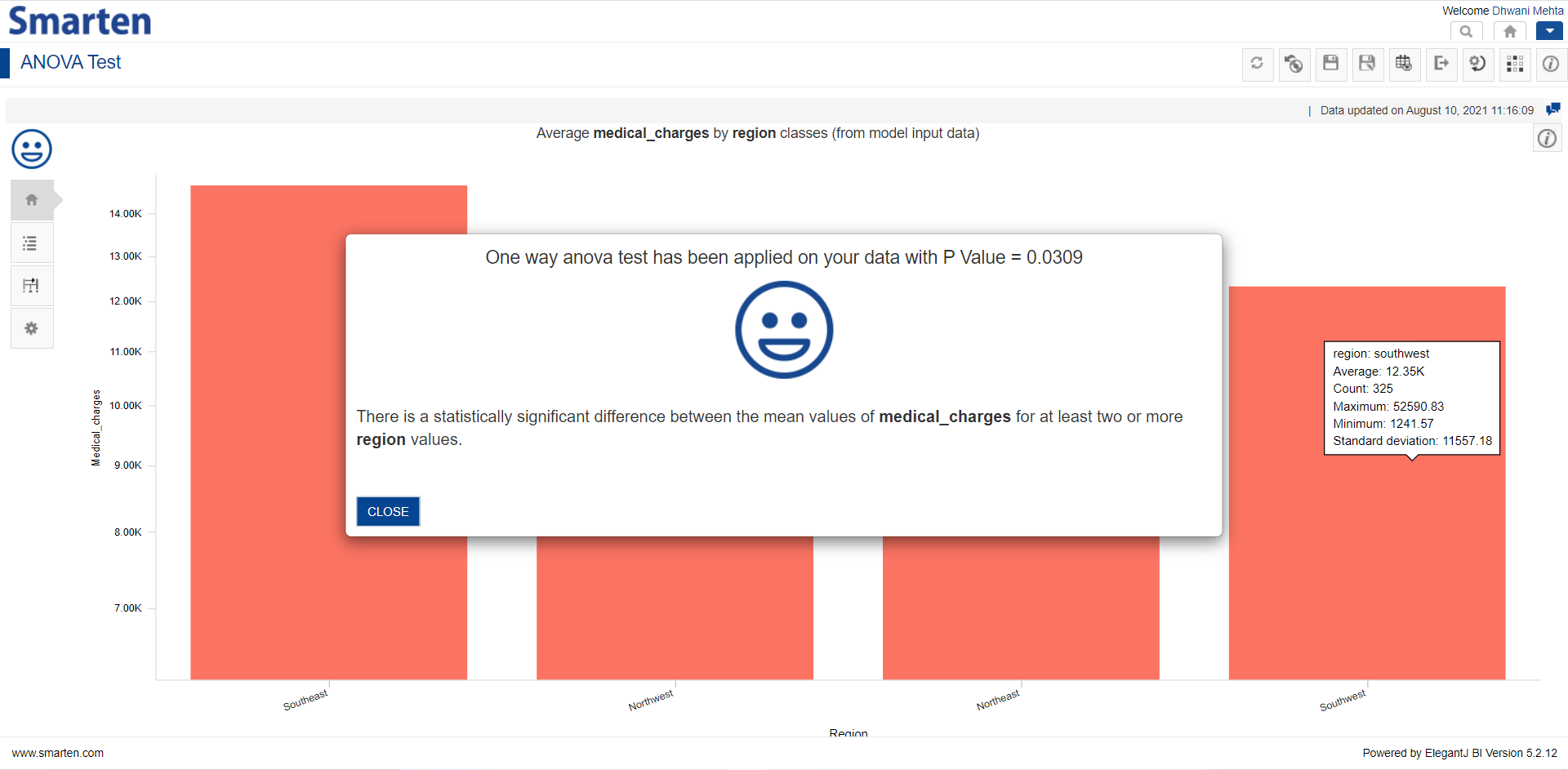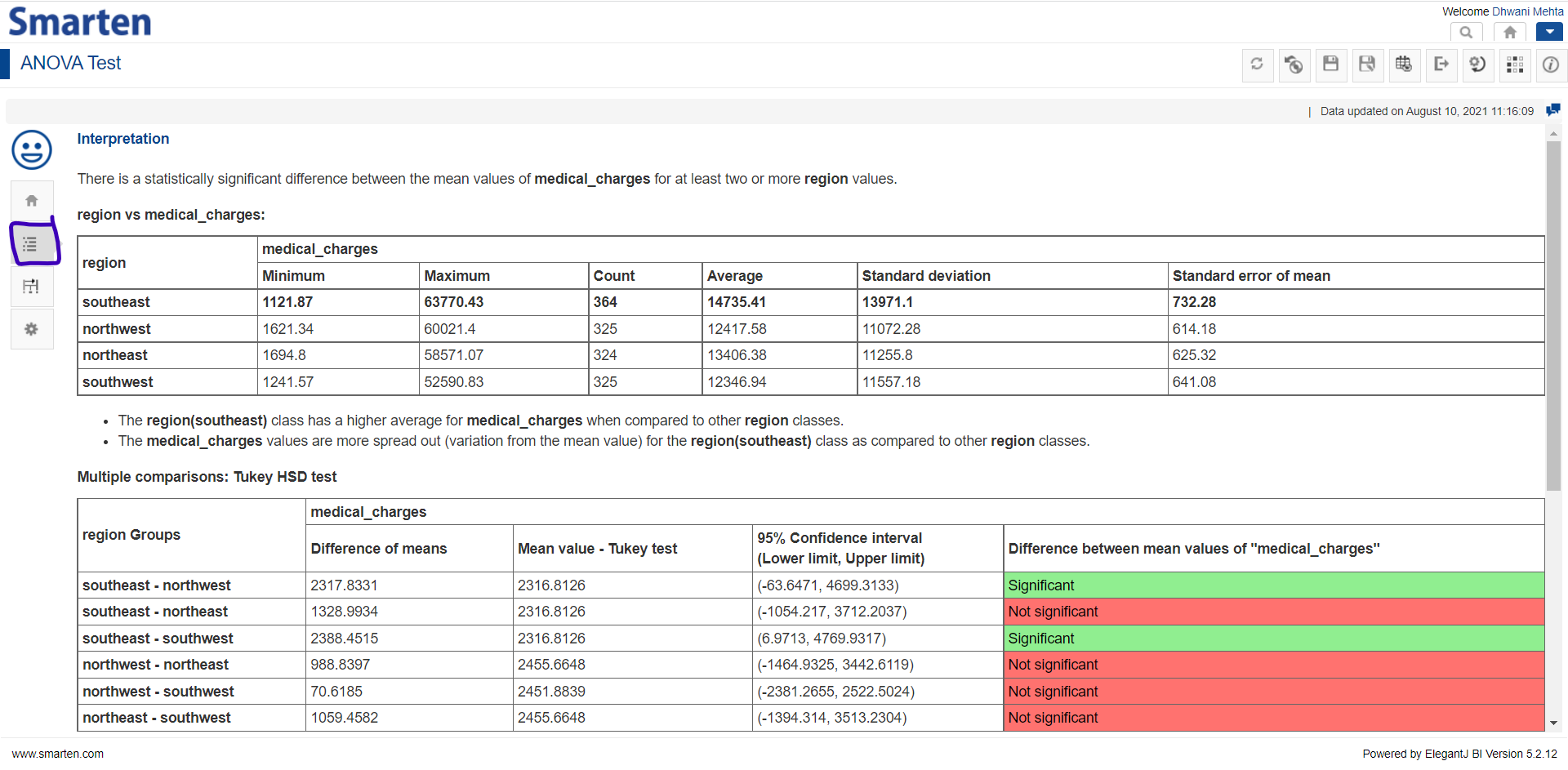1.     It's hard to tell the truth without statistics!

Statistical analysis is a crucial component of data analytics useful in interpreting the behavior of data and uncovering the patterns and trends underlying it. It assists in describing the nature of the data to be analyzed and exploring the relationships among the data attributes. Furthermore, the tests performed for statistical analysis proves (or disproves) the validity of the statistical model. There is a wide range of statistical tests which can be performed and the choice of which relies upon the structure and nature of the data and the variable type. This post focuses on One-Way Anova test and inferring data using this test utilizing Smarten Augmented Analytics.

2.      A hypothesis is an idea which can be tested!

A Hypothesis test is a statistical test that is used to determine whether there is enough evidence in a sample of data to infer that a certain condition is true for the entire population. The foremost step in any hypothesis test is to define the Null hypothesis (H₀) and Alternate hypothesis (H₁) and then perform the respective test which will either reject the defined Null hypothesis and accept the alternate hypothesis, or accept the null hypothesis and reject the alternate hypothesis. Null hypothesis (H₀) is an argument we believe to be true even before we collect any data. Alternate hypothesis (H₁) is an argument we want to prove to be true.

3.     The best way to understand is by an example!

Let’s consider that we have medical data for various regions of a country and want to examine whether those different regions (i.e., northeast, southeast, northwest, southwest) have more or less equivalent medical costs or does the medical cost is region specific and there is much difference in the prices of medicines based upon different geographic locations. Now this can be tested using Hypothesis testing by defining out hypothesis as follows:

Null hypothesis (H₀): These is not much difference in medical prices based upon region.

Alternate hypothesis (H₁): There is significant difference in medical prices based upon region.

So, on performing the statistical tests on our medical data, we get to either accept or reject the null hypothesis. In order to quantify this identification for our hypothesis, we need to determine the significance level (alpha) which is a probability of rejecting our null hypothesis, provided it's actually true. Any statistical test we perform to make conclusions for our hypothesis, calculates a p-value, which describes whether the outcome of the statistical test is statistically significant.

Low p-value: Your sample results are not consistent with a null hypothesis.

High p-value: Your sample results are consistent with a true null hypothesis.

This implies that, smaller the p-value, the sample is inconsistent with the null hypothesis and one can reject the null hypothesis.

4.    One-Way Anova Test

ANOVA stands for Analysis of Variance. This test tells us if there is a statistical difference between the means of three or more independent groups. One-Way Anova Test can be used when we have one categorical independent variable with at least 3 different categories (e.g., region variable with northeast, southeast, northwest and southwest categories) and one quantitative dependent variable (e.g., medical charges).

5.    Performing One-Way Anova Test using Smarten

Having an understanding about the One-Way ANOVA test and knowing its terminologies broadly, let’s dive swiftly to performing this test straightforwardly using Smarten Augmented Analytics!

5.1.        Create a fresh New Smarten Insight

Go to New -> SmartenInsight Menu in the dropdown provided in the top right corner of the Smarten Dashboard.Creating a new Smarten Insight

5.2.        Select data to perform ANOVA test upon and click NEXTSearch and Select dataset to Perform ANOVA Test upon

5.3.        Perform data pre-processing steps i.e., sampling and filtering if required and click NEXTData Pre-Processing using Smarten

5.4.        Handle Outliers and Missing Values if required and click NEXTHandling Outliers and Missing Values using Smarten

5.5.      What do you want to do?

After performing all the data processing and handling steps, Smarten asks users to perform their respective algorithmic technique to proceed with. Let’s select the Hypothesis testing.Ask Smarten to Perform Hypothesis testing

5.6.      Smarten knows our objective and automates performance of corresponding best fit hypothesis test

As described earlier, ANOVA test is used to analyze if there is statistically significant difference among 3 or more groups and this test can be used when we have one categorical independent variable with at least 3 different categories (e.g., region variable with northeast, southeast, northwest and southwest categories) and one quantitative dependent variable (e.g., medical charges). Let’s select our variables accordingly and click NEXT!Variable selection for Hypothesis testing

6.     And here we go! One-Way Anova test performed

One-Way ANOVA test performed and the corresponding visualization allows users get an easy insight upon how the group means vary.One-Way Anova Test using Smarten

7.   Interpretation of Anova Test

In order to understand the insights from the hypothesis performed, navigate to the Interpretation tab to understand the variability among the chosen variables in simple language.Basic Interpretation of One-Way Anova test using Smarten

8.     Model Summary to obtain technical details about One-Way Anova test

In order to obtain a technical understanding about the variation in variables and hypothesis acceptance, the Model Summary tab is provided.Model Summary for One Way Anova test - Smarten

9.    It’s a wrap!

It can be prominently inferred from the results, interpretation and model summary that there is significant difference among the medical charges based upon region with region southeast-southwest and southeast-northwest exhibiting statistically significant difference in their mean values. It can be stated henceforth that Healthcare costs vary widely by region! The interested groups can furthermore carry out operations to focus upon the root cause for this variation which might be the competition of medical services in a specific region, the number of insurers in the region and the availability of services or physicians in that area. It is as straight as an arrow to perform Anova Test with the different parameters and use-cases to understand the variability among target categorical variable based upon a numeric dependent variable utilizing Smarten Augmented Analytics!